Courses

# Test: First Law Applied to Flow Process

## 10 Questions MCQ Test Thermodynamics | Test: First Law Applied to Flow Process

Description
This mock test of Test: First Law Applied to Flow Process for Mechanical Engineering helps you for every Mechanical Engineering entrance exam. This contains 10 Multiple Choice Questions for Mechanical Engineering Test: First Law Applied to Flow Process (mcq) to study with solutions a complete question bank. The solved questions answers in this Test: First Law Applied to Flow Process quiz give you a good mix of easy questions and tough questions. Mechanical Engineering students definitely take this Test: First Law Applied to Flow Process exercise for a better result in the exam. You can find other Test: First Law Applied to Flow Process extra questions, long questions & short questions for Mechanical Engineering on EduRev as well by searching above.
QUESTION: 1

### Neglecting changes in potential and kinetic energies, the shaft work during a steady flow process is given by

Solution:

Work in steady flow process  =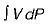Work in non-flow process =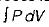QUESTION: 2

### Consider the following statements about steady flow process: 1. The rate of flow of mass energy across the control surface are constant. 2. Thermodynamic properties vary along space as well as time coordinates. 3. Any thermodynamic property will have a fixed value at a particular location and will not alter with time. Which of the above are correct?

Solution:

Thermodynamic properties may vary along space coordinates but do not vary with time.

QUESTION: 3

### Consider following comparative points of S.F.E.E with Bernoulli's equation: 1. These two have several terms in common. 2. Both Bernoulli’s equation and S.F.E.E is valid for viscous compressible fluids. 3. The Bernoulli’s equation is a special limiting case of S.F.E.E Which of the above is/are correct?

Solution:

Bernoulli’s equation valid for frictionless incompressible fluids. S.F.E.E. valid for viscous compressible fluids.

QUESTION: 4

Neglecting changes in kinetic and potential energies, the identity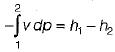for the shaft work during a steady flow process is valid for

Solution:
QUESTION: 5

The term Δh in a control volume equation Q - W = Δh

Solution:

S.F.E.E: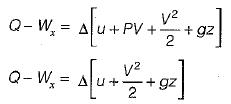Δh accounts for internal energy and pressure forces

QUESTION: 6

A key concept in analyzing the filling of an evacuated tank is

Solution:
QUESTION: 7

Match List-I (Devices) with List-ll (Thermodynamics equations) and select the correct answer using the codes given below the lists: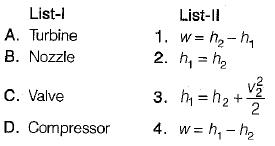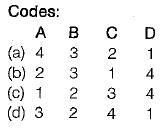Solution:
QUESTION: 8

If steam is throttled its

Solution:

If steam is throttled , its enthalpy remains constant and pressure drop takes place

QUESTION: 9

Which one of the following expression is correct for reversible work done by the system (steady flow) between state 1 and 2 ?

Solution:
QUESTION: 10

Compressed air coming out from a punctured football

Solution: